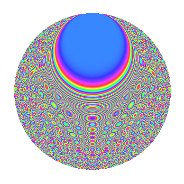# Properties

 Label 160.6.uLevel 160 Weight 6 Character orbit u Rep. character $$\chi_{160}(43,\cdot)$$ Character field $$\Q(\zeta_{8})$$ Dimension 472 Sturm bound 144

# Related objects

## Defining parameters

 Level: $$N$$ $$=$$ $$160 = 2^{5} \cdot 5$$ Weight: $$k$$ $$=$$ $$6$$ Character orbit: $$[\chi]$$ $$=$$ 160.u (of order $$8$$ and degree $$4$$) Character conductor: $$\operatorname{cond}(\chi)$$ $$=$$ $$160$$ Character field: $$\Q(\zeta_{8})$$ Sturm bound: $$144$$

## Dimensions

The following table gives the dimensions of various subspaces of $$M_{6}(160, [\chi])$$.

Total New Old
Modular forms 488 488 0
Cusp forms 472 472 0
Eisenstein series 16 16 0

## Trace form

 $$472q - 4q^{2} - 4q^{3} - 4q^{5} - 8q^{6} - 496q^{8} + O(q^{10})$$ $$472q - 4q^{2} - 4q^{3} - 4q^{5} - 8q^{6} - 496q^{8} + 1064q^{10} - 8q^{11} + 1580q^{12} - 4q^{13} + 256q^{14} - 8q^{15} - 8q^{16} + 124q^{18} - 4720q^{19} - 1656q^{20} - 8q^{21} + 5580q^{22} - 16160q^{24} - 4q^{25} - 8q^{26} - 976q^{27} + 4092q^{28} + 63488q^{30} + 25576q^{32} - 8q^{33} + 27640q^{34} + 17272q^{35} - 8q^{36} - 4q^{37} - 2860q^{38} - 31184q^{40} - 8q^{41} + 59540q^{42} - 1316q^{43} + 9680q^{44} - 4q^{45} - 8q^{46} - 8q^{47} - 201944q^{48} - 1018024q^{49} - 132q^{50} + 20872q^{51} - 128136q^{52} - 4q^{53} - 154280q^{54} + 110044q^{55} - 129368q^{56} - 8q^{57} + 97564q^{58} + 4092q^{60} - 96168q^{61} - 103160q^{62} + 134456q^{63} - 234960q^{64} - 8q^{65} + 109992q^{66} - 89260q^{67} - 155832q^{68} + 1944q^{69} - 132540q^{70} - 143848q^{71} - 311016q^{72} - 8q^{73} + 968q^{75} - 12488q^{76} + 67224q^{77} + 747632q^{78} + 376632q^{80} - 67796q^{82} - 126444q^{83} + 134456q^{84} - 4q^{85} - 91048q^{86} + 564360q^{87} + 167304q^{88} - 725172q^{90} - 8q^{91} + 118444q^{92} + 968q^{93} + 238240q^{94} + 304152q^{96} - 8q^{97} + 971064q^{98} + O(q^{100})$$

## Decomposition of $$S_{6}^{\mathrm{new}}(160, [\chi])$$ into newform subspaces

The newforms in this space have not yet been added to the LMFDB.

## Hecke characteristic polynomials

There are no characteristic polynomials of Hecke operators in the database